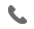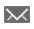•+44 7459 302492info@uplatz.com
• Register

# VLookup Function with Exact Match | MS Excel

Details

VLOOKUP function in MS Excel is a function which is used to retrieve data from a specific column in an Excel table. The letter ‘V’ in VLOOKUP stands for “Vertical” LOOKUP and all the Lookup values which have been retrieved using this function must appear in the first column of the table. Its syntax is:
=VLOOKUP(value, table, col_index, [range_lookup])

The VLOOKUP function in Excel supports both the “Exact Match” and the “Approx Match”. The VLOOKUP function with exact match helps users in finding the exact match data for the particular column in an excel spreadsheet.

Category
Tags